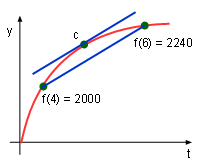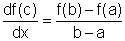Ch 4. The Mean Value Theorem Multimedia Engineering Math Maximum & Minimum Rolle'sTheorem Mean ValueTheorem MonotonicFunctions First DerivativeTest Concavity &Inflection SecondDerivative Test
 Chapter 1. Limits 2. Derivatives I 3. Derivatives II 4. Mean Value 5. Curve Sketching 6. Integrals 7. Inverse Functions 8. Integration Tech. 9. Integrate App. 10. Parametric Eqs. 11. Polar Coord. 12. Series Appendix Basic Math Units Search eBooks Dynamics Fluids Math Mechanics Statics Thermodynamics Author(s): Hengzhong Wen Chean Chin Ngo Meirong Huang Kurt Gramoll ©Kurt GramollMATHEMATICS - CASE STUDY SOLUTIONDriving Process (Unit: mile) Peter is driving on on a road where the speed limit is 65 mi/hr. By 4 p.m. he has drove 2000 miles and by 6 p.m. his total trip mileage goes up to 2240 miles. Was Peter speeding during in this period of time? Let f(t) be the car's position at time t. By 4 p.m. he traveled 2000 miles, so f(4) = 2000 By 6 p.m. he traveled 2240 miles, so f(6) = 2240 The mean value theorem gives thatIn this case, the mean value on interval [4, 6] is      df(c)/dx = (f(6) - f(4))/(6 - 4) = (2240 - 2000)/2 = 120 Since f(t) is a position function, the derivative of it is velocity. In other words, the above equation means that there is a time c in the interval of 4 and 6 such that the velocity is 120 mi/hr. Therefore, Peter exceeded the speed limit.